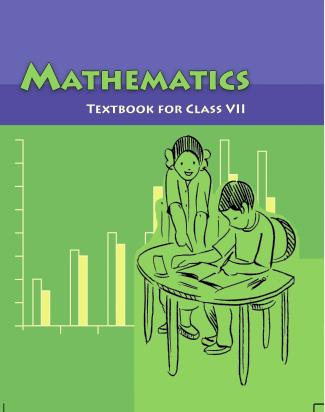1. /
2. CBSE
3. /
4. Class 07
5. /
6. Mathematics
7. /
8. NCERT Solutions for Class...

# NCERT Solutions for Class 7 Maths Exercise 8.1### myCBSEguide App

Download the app to get CBSE Sample Papers 2023-24, NCERT Solutions (Revised), Most Important Questions, Previous Year Question Bank, Mock Tests, and Detailed Notes.

NCERT solutions for Maths Comparing Quantities## NCERT Solutions for Class 7 Maths Comparing Quantities

###### Question 1. Find the ratio of:

(a) Rs. 5 to 50 paise

(b) 15 kg to 210 g

(c) 9 m to 27 cm

(d) 30 days to 36 hours

To find ratios, both quantities should be in same unit.

(a) Rs. 5 to 50 paise

{tex} \Rightarrow {/tex} 5 x 100 paise to 50 paise

[{tex}\because {/tex}Rs. 1 = 100 paise]

{tex} \Rightarrow {/tex} 500 paise to 50 paise

Thus, the ratio is = {tex}\frac{{500}}{{50}} = \frac{{10}}{1}{/tex} = 10 : 1

(b) 15 kg to 210 g

{tex} \Rightarrow {/tex} 15 x 1000 g to 210 g [ {tex}\because {/tex} 1 kg = 1000 g]

{tex} \Rightarrow {/tex} 15000 g to 210 g

Thus, the ratio is = {tex}\frac{{15000}}{{210}} = \frac{{500}}{7}{/tex} = 500 : 7

(c) 9 m to 27 cm

{tex} \Rightarrow {/tex} 9 x 100 cm to 27 cm [ {tex}\because {/tex} 1 m = 100 cm]

{tex} \Rightarrow {/tex} 900 cm to 27 cm

Thus, the ratio is = {tex}\frac{{900}}{{27}} = \frac{{100}}{3}{/tex} = 100 :3

(d) 30 days to 36 hours

{tex} \Rightarrow {/tex} 30 x 24 hours to 36 hours [ {tex}\because {/tex} 1 day = 24 hours]

{tex} \Rightarrow {/tex} 720 hours to 36 hours

Thus, the ratio is = {tex}\frac{{720}}{{36}} = \frac{{20}}{1}{/tex} = 20 : 1

NCERT Solutions for Class 7 Maths Exercise 8.1

###### Question 2.In a computer lab, there are 3 computers for every 6 students. How many computers will be needed for 24 students?

(a) 0.65 = {tex}\frac{{65}}{{100}} \times 100\% {/tex} = 65%

(b) 0.02 = {tex}\frac{2}{{100}} \times 100\% {/tex} = 2%

NCERT Solutions for Class 7 Maths Exercise 8.1

###### Question 3.Population of Rajasthan = 570 lakhs and population of U.P. = 1660 lakhs. Area of Rajasthan = 3 lakh {tex}k{m^2}{/tex}and area of U.P. = 2 lakh{tex}k{m^2}{/tex}.

(i) How many people are there per km2 in both states?

(ii) Which state is less populated?

(i) People present per {tex}k{m^2}{/tex}= {tex}\frac{{{\text{Population}}}}{{{\text{Area}}}}{/tex}

In Rajasthan = {tex}\frac{{570{\text{ lakhs}}}}{{{\text{3 lakhs per k}}{{\text{m}}^2}}}{/tex} = 190 people {tex}k{m^2}{/tex}

In U.P. = {tex}\frac{{{\text{1660 lakhs}}}}{{{\text{2 lakh per k}}{{\text{m}}^2}}}{/tex} = 830 people per {tex}k{m^2}{/tex}

(ii) Rajasthan is less populated.

## NCERT Solutions for Class 7 Maths Exercise 8.1

NCERT Solutions Class 7 Maths PDF (Download) Free from myCBSEguide app and myCBSEguide website. Ncert solution class 7 Maths includes text book solutions from Class 7 Maths Book . NCERT Solutions for CBSE Class 7 Maths have total 15 chapters. 7 Maths NCERT Solutions in PDF for free Download on our website. Ncert Maths class 7 solutions PDF and Maths ncert class 7 PDF solutions with latest modifications and as per the latest CBSE syllabus are only available in myCBSEguide.

## CBSE app for Students

To download NCERT Solutions for Class 7 Maths, Social Science Computer Science, Home Science, Hindi English, Maths Science do check myCBSEguide app or website. myCBSEguide provides sample papers with solution, test papers for chapter-wise practice, NCERT solutions, NCERT Exemplar solutions, quick revision notes for ready reference, CBSE guess papers and CBSE important question papers. Sample Paper all are made available through the best app for CBSE students and myCBSEguide website.### Test Generator

Create question paper PDF and online tests with your own name & logo in minutes.### myCBSEguide

Question Bank, Mock Tests, Exam Papers, NCERT Solutions, Sample Papers, Notes

### 1 thought on “NCERT Solutions for Class 7 Maths Exercise 8.1”

1. Nice Web site